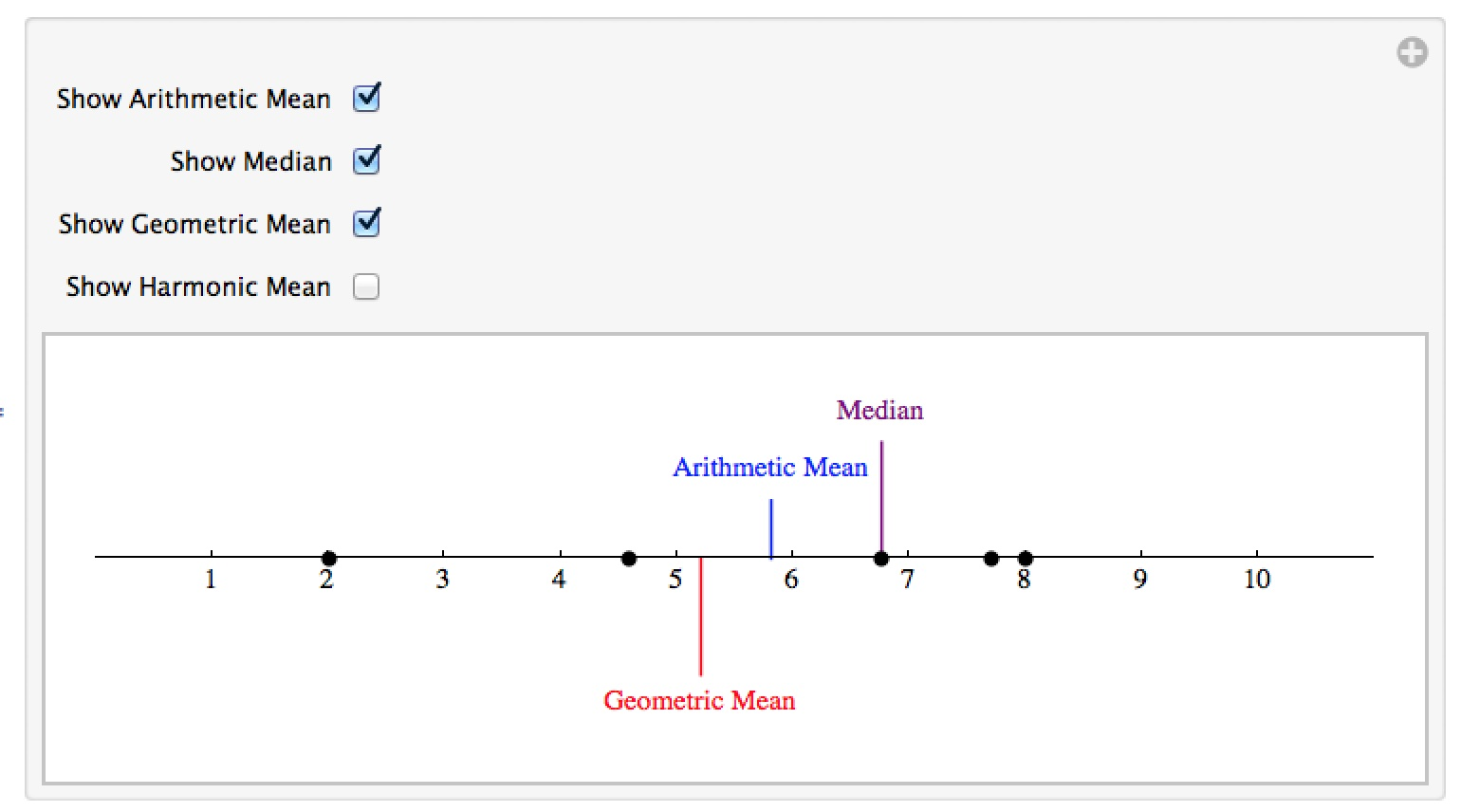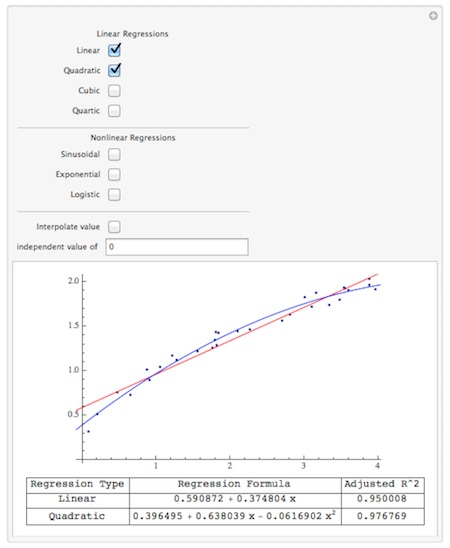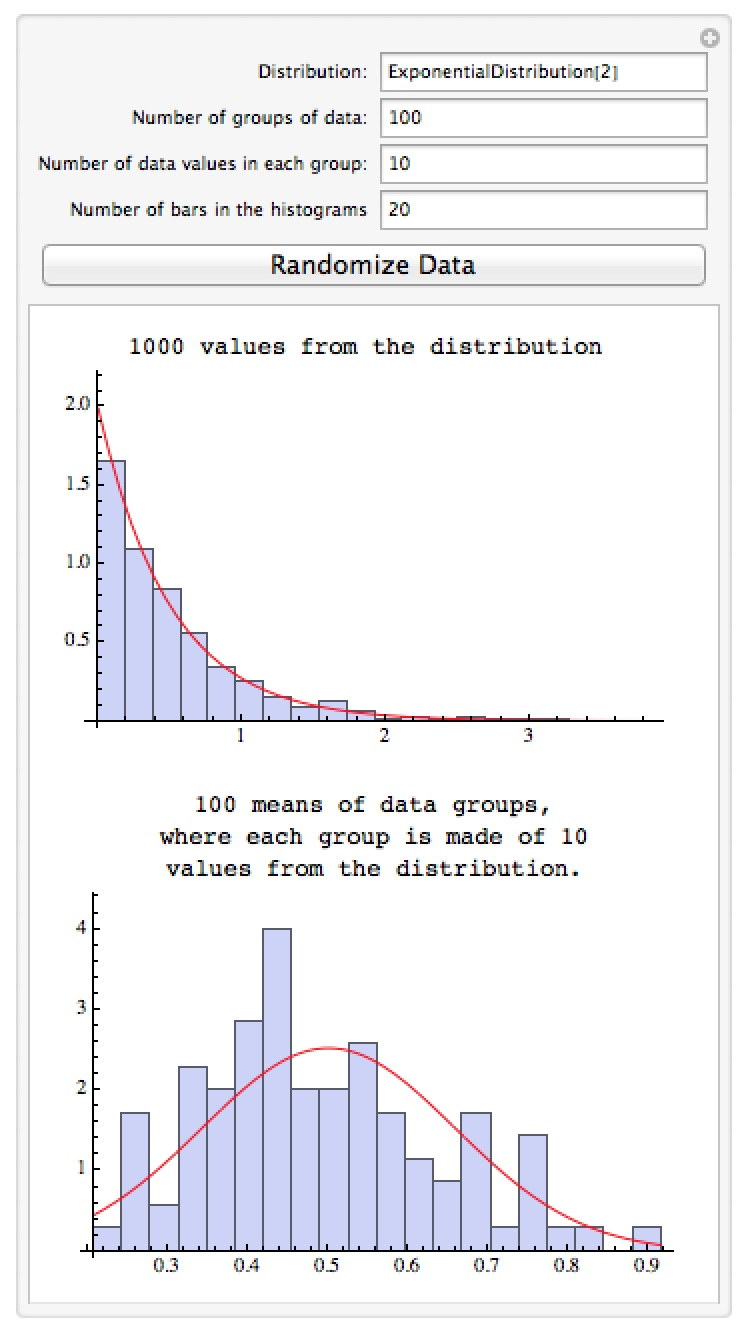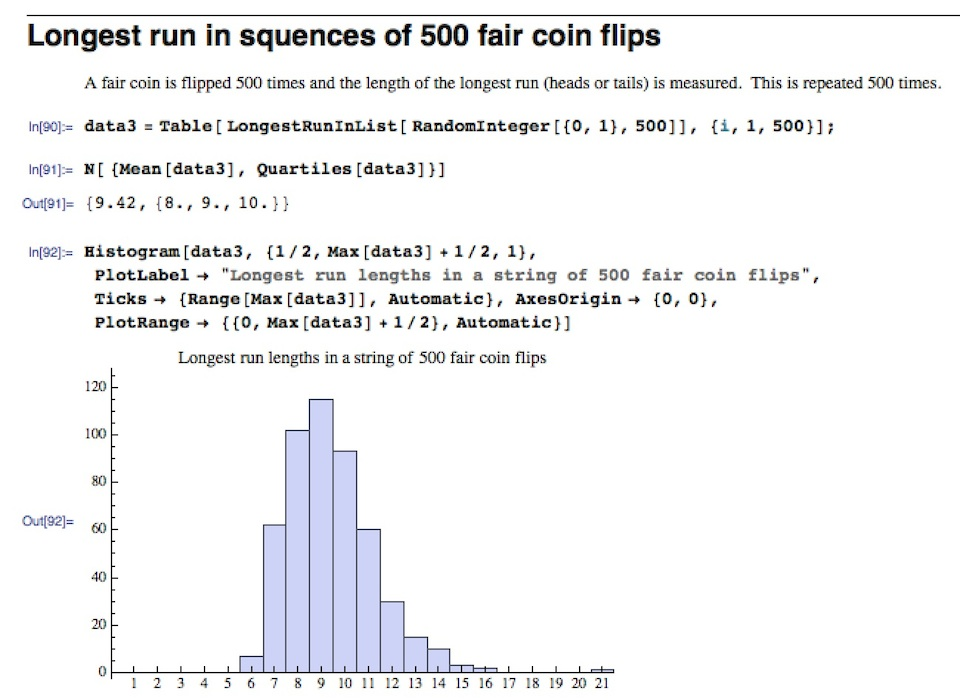# Mathematica Notebooks for Probability and Statistics

Important Note: The links for the notebooks open a new window or tab with a Google Drive page – the current settings for our homepages won’t allow me to host mathematica notebooks locally.

## Measures of Central Tendency

This notebook contains an animation which explores 4 different measures of central tendency – the mean, median, geometric mean, and harmonic mean.  You can add additional data points to the number line by command-clicking on the line, and each point can be slid up and down the line with the measures of central tendency automatically updating (which is useful for demonstrating how differently measures like the mean and median react to changing a single value).## Linear Regression

This notebook contains a command called RegressionAnalysis.  Given a list of data points data, RegressionAnalysis[ datax ] creates a manipulation which lets you explore common linear and non-linear regressions, view the adjusted R^2 values for them, and interpolate values.  I wrote this as a first attempt at duplicating some of the functionality of the stat package JMP within Mathematica; we’ve since moved from JMP to Mathematica for doing basic statistics in our Algebra for the Sciences course (using the LinearModelFit command rather than this code).## The Central Limit Theorem

This notebook lets you explore the Central Limit Theorem from probability – the theorem that says no matter what distribution you draw from, if you take averages of measurements the averages tend to be normally distributed (the larger the number of points being averaged, the closer you should get to the normal distribution).  The manipulation lets you select the initial distribution the data will come from, the number of data points per group for the average, and the number of groups to compute an average for.  For example in the picture below the 1000 data points are drawn from the highly non-normal exponential distribution; below that is the distribution of 100 averages (each average being of 10 points) from the same distribution.  Even though the original distribution doesn’t come close to a bell curve and 10 isn’t a large number of values to average, the distribution of the averages looks a lot more like a bell curve (typically you want to average 30 or more points to really get close to the bell curve).